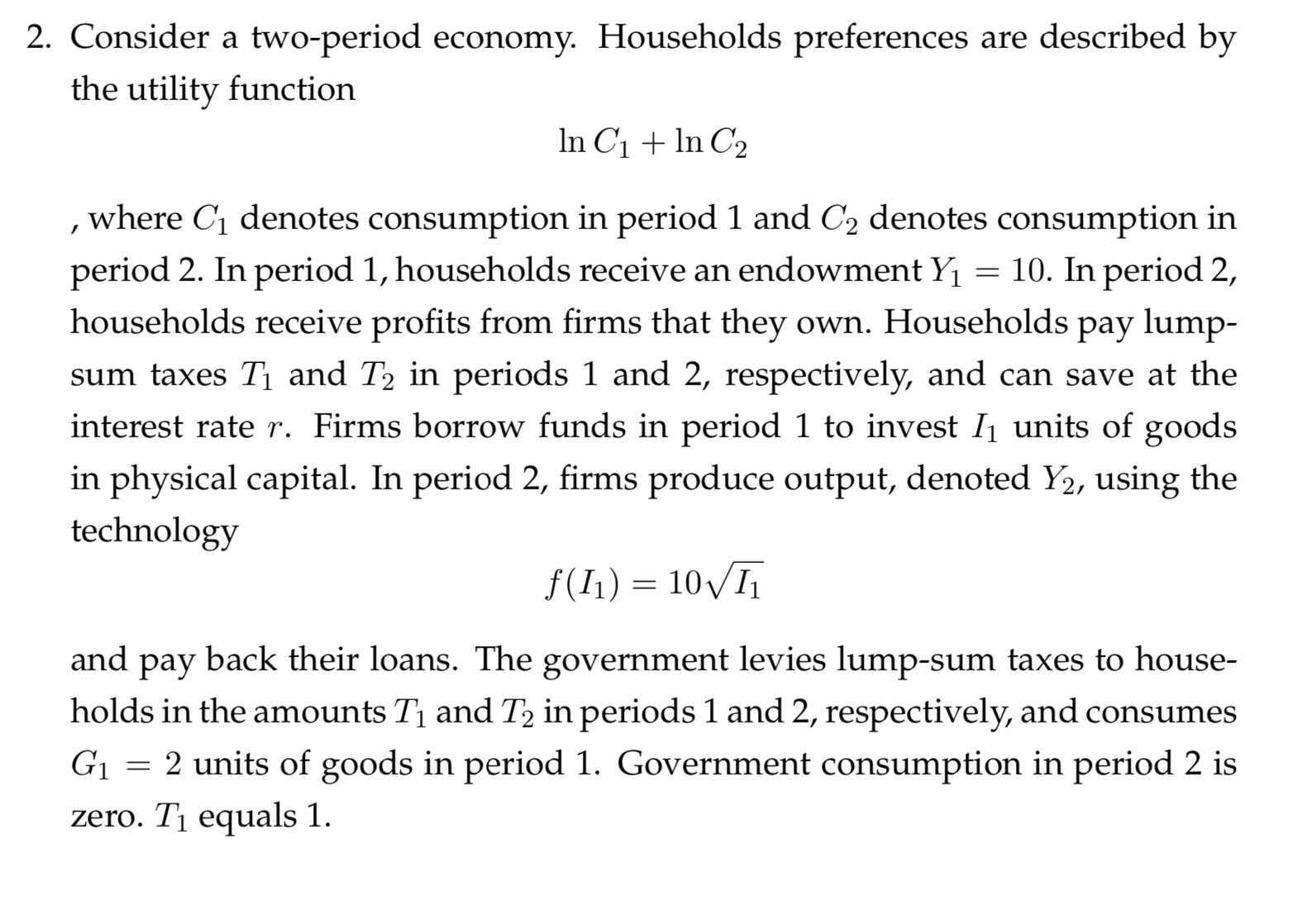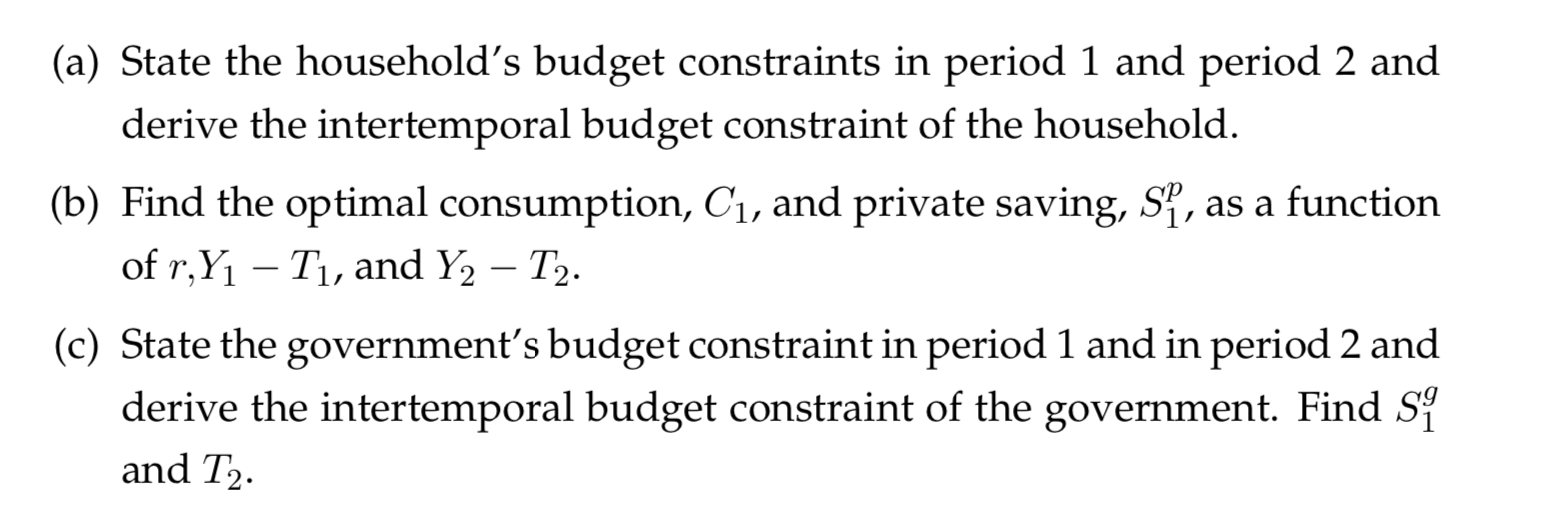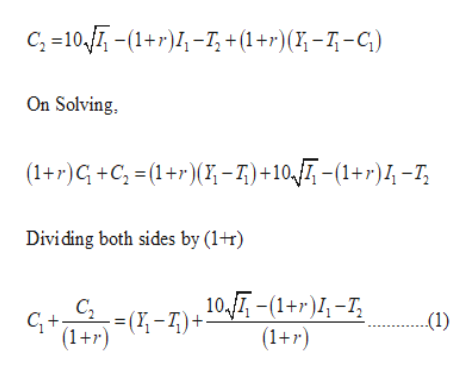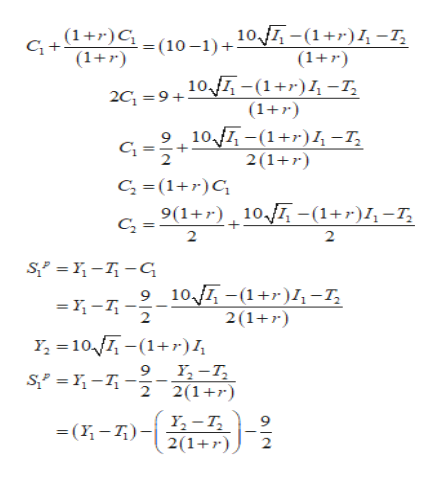# 2. Consider a two-period ecornomy. Households preferences are described bythe utility functionIn C1n C2, where C1 denotes consumption in period 1 and C2 denotes consumption inperiod 2. In period 1, households receive an endowment Yhouseholds receive profits from firms that they own. Households pay lump-= 10. In period 2,sum taxes Ti and T2 in periods 1 and 2, respectively, and can save at theinterest rate r. Firms borrow funds in period 1 to invest I1 units of goodsin physical capital. In period 2, firms produce output, denoted Y2, using thetechnologyf(I1) 10Iandback their loans. The government levies lump-sum taxes to house-рayholds in the amounts Ti and T2 in periods 1 and 2, respectively, and consumesG2 units of goods in period 1. Government consumption in period 2 iszero. T1 equals 1. (a) State the household's budget constraints in period 1 and period 2 andderive the intertemporal budget constraint of the household.(b) Find the optimal consumption, Ci, and private saving, S, as a functionof r,Y1 Ti, and Y2 T2.(c) State the government's budget constraint in period 1 and in period 2 andderive the intertemporal budget constraint of the government. Find Siand T2

Question
357 views

I need help with a-chelp_outlineImage Transcriptionclose2. Consider a two-period ecornomy. Households preferences are described by the utility function In C1n C2 , where C1 denotes consumption in period 1 and C2 denotes consumption in period 2. In period 1, households receive an endowment Y households receive profits from firms that they own. Households pay lump- = 10. In period 2, sum taxes Ti and T2 in periods 1 and 2, respectively, and can save at the interest rate r. Firms borrow funds in period 1 to invest I1 units of goods in physical capital. In period 2, firms produce output, denoted Y2, using the technology f(I1) 10I and back their loans. The government levies lump-sum taxes to house- рay holds in the amounts Ti and T2 in periods 1 and 2, respectively, and consumes G 2 units of goods in period 1. Government consumption in period 2 is zero. T1 equals 1. fullscreenhelp_outlineImage Transcriptionclose(a) State the household's budget constraints in period 1 and period 2 and derive the intertemporal budget constraint of the household. (b) Find the optimal consumption, Ci, and private saving, S, as a function of r,Y1 Ti, and Y2 T2. (c) State the government's budget constraint in period 1 and in period 2 and derive the intertemporal budget constraint of the government. Find Si and T2 fullscreen
check_circle

Step 1

(a) Household’s budget constraint in period 1 is:

C1 + S1p = Y1 – T1

Household’s budget constraint in period 2 and the intertemporal budget constraint of the household is:help_outlineImage TranscriptioncloseC, =10 1+r)-I,+(1+r)(% -7-C) On Solving (1+r)G+C; =(1+r)(;-7)+10T, -(1+r)4-7;| Dividing both sides by (1+r) 10,-1+r)-T, (1+r) CL (1) (1+r) (-7)+ fullscreen
Step 2

(b) Now to find the optimal consumption level we will equate the slope of budget constraint (BC) with the marginal rate of substitution (MRS).

Slope of BC = (1+r)

MRS = U’C1 / U’C2

= (1/C1) / (1/C2)

= C2 / C1

Equating the ...help_outlineImage Transcriptionclose= (10-1)+10,-(1+r)4-7; (1r) G+1+r)C (1+ 10-(1+r)-I, (1+r) 9 10 -(1)4-I, 2C 9+ 2(1+r C =(1+r)C 9(1+r) 10-(1+r)I,-I, 2 2 S - G 9 10-(1)I,-I, 2 2(1+ Y 10 (1r)1, 9 Y-T 2(1 2 Y,-T 2(1r (- fullscreen

### Want to see the full answer?

See Solution

#### Want to see this answer and more?

Solutions are written by subject experts who are available 24/7. Questions are typically answered within 1 hour.*

See Solution
*Response times may vary by subject and question.
Tagged in